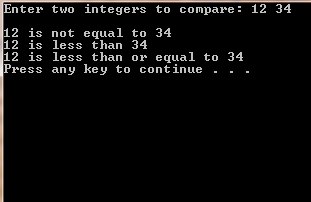# C++ Code to Find Various Relations Between two Numbers Entered (greater than, equal to, smaller than, greater than equal to & smaller than equal to)

/*In this example, we are write a program which is able to compare two numbers in different ways.
Here we have used the “if” conditional statement, & relational operators to find various comparision,
relations between various numbers, such as greater than, smaller than, equal, not equal, greater than on equal, & smaller than & equal*/

//Comparing two numbers
//Using if statements, relational operators

#include <iostream>
#include<stdlib.h>
//For system("pause") function
using namespace std;

int main()
{
int m;
int n;

cout << "Enter two integers to compare: ";
cin >> m >> n;
// user enters two integers
cout<<endl;

//Actual comparision of two numbers starts here

if ( m == n )
cout << m << " is equal to " << n << endl;

if ( m != n )
cout << m << " is not equal to " << n << endl;

if ( m < n )
cout << m << " is less than " << n << endl;

if ( m > n )
cout << m<< " is greater than " << n << endl;

if ( m <= n)
cout << m << " is less than or equal to "<< n << endl;

if ( m >= n )
cout << m << " is greater than or equal to "<< n << endl;
system("pause");
return 0;
}

ScreenShots: